Function Repository Resource:

# MatrixPencilSolve

Find the null values and vectors for the pencil of a set of square matrices

Contributed by: Daniel Lichtblau
 ResourceFunction["MatrixPencilSolve"][{m0,m1,…,mn}] finds the generalized eigenvalues λ and corresponding vectors v for which the matrix m0+λm1+λ2m2+…λnmn has a nontrivial null space.

## Details and Options

The matrix inputs must be square and of the same dimensions.
The result is a list of lists of the form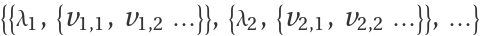.

## Examples

### Basic Examples (3)

Form some random real-valued matrices:

 In:=Find the null-valued vectors for the pencil of these matrices:

 In:=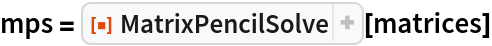Out=Check that the first of these value/vector pairs satisfies the necessary equation:

 In:=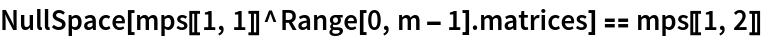Out=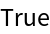### Scope (1)

MatrixPencilSolve will handle exact as well as approximate input matrices:

 In:=Out=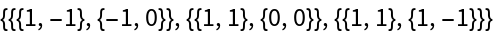In:=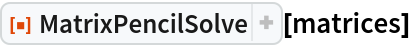Out=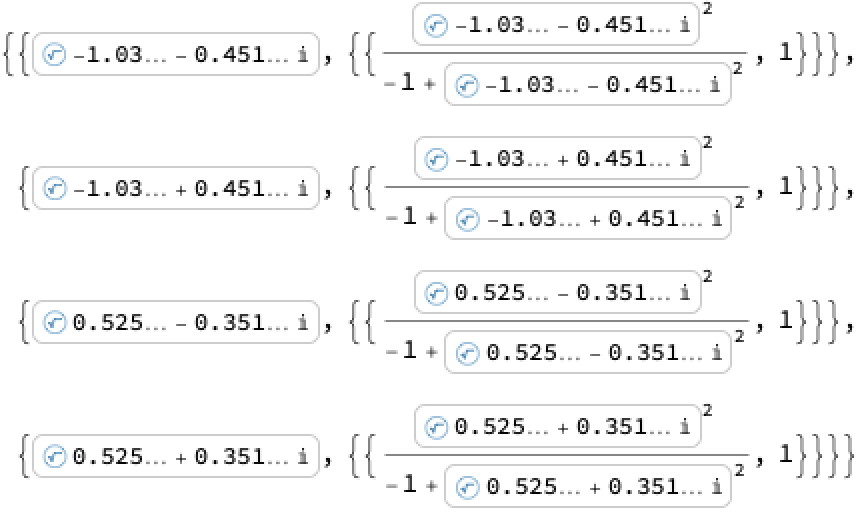### Properties and Relations (2)

MatrixPencilSolve with one argument is equivalent to obtaining vectors from NullSpace, with λ having no value:

 In:=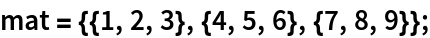In:=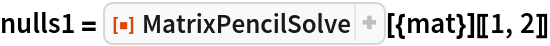Out=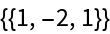In:=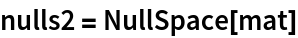Out=MatrixPencilSolve with two matrices is equivalent to the two-argument form of Eigensystem, with the second matrix negated and the result transposed:

 In:=Out=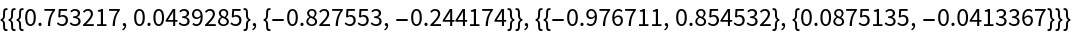In:=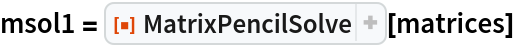Out=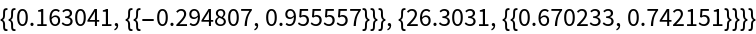In:=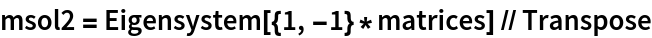Out=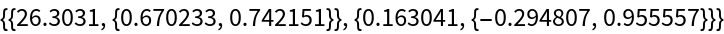## Requirements

Wolfram Language 11.3 (March 2018) or above

## Version History

• 1.0.0 – 28 February 2019